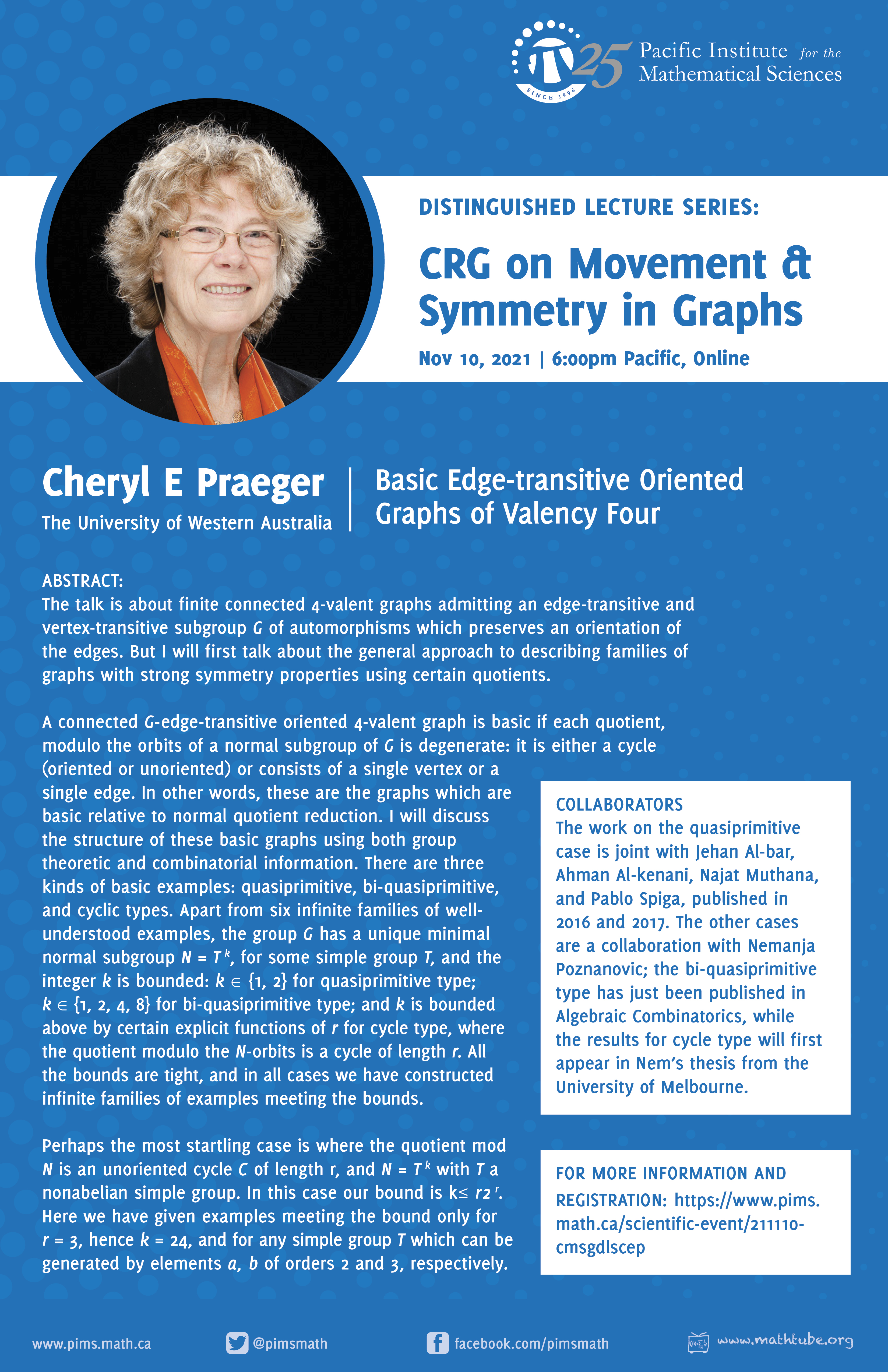## CRG on Movement and Symmetry in Graphs Distinguished Lecture Series: Cheryl E Praeger

• Date: 11/10/2021
• Time: 18:00
Lecturer(s):
Cheryl E Praeger, University of Western Australia
Location:

Online

Topic:

Basic edge-transitive oriented graphs of valency four

Description:

The talk is about finite connected 4-valent graphs admitting an edge-transitive and vertex-transitive subgroup $$G$$ of automorphisms which preserves an orientation of the edges. But I will first talk about the general approach to describing families of graphs with strong symmetry properties using certain quotients.

A connected $$G$$-edge-transitive oriented 4-valent graph is basic if each quotient, modulo the orbits of a normal subgroup of $$G$$ is degenerate: it is either a cycle (oriented or unoriented) or consists of a single vertex or a single edge. In other words, these are the graphs which are basic relative to normal quotient reduction. I will discuss the structure of these basic graphs using both group theoretic and combinatorial information. There are three kinds of basic examples: quasiprimitive, bi-quasiprimitive, and cyclic types. Apart from six infinite families of well-understood examples, the group $$G$$ has a unique minimal normal subgroup $$N=T^k$$, for some simple group $$T$$, and the integer $$k$$ is bounded: $$k\in \left\{1, 2\right\}$$ for quasiprimitive type; $$k \in \left\{1, 2, 4, 8\right\}$$ for bi-quasiprimitive type; and $$k$$ is bounded above by certain explicit functions of $$r$$ for cycle type, where the quotient modulo the $$N$$-orbits is a cycle of length $$r$$. All the bounds are tight, and in all cases we have constructed infinite families of examples meeting the bounds.

Perhaps the most startling case is where the quotient mod $$N$$ is an unoriented cycle $$C$$ of length $$r$$, and $$N = T^k$$ with $$T$$ a nonabelian simple group. In this case our bound is $$k\leq r2^r$$. Here we have given examples meeting the bound only for $$r=3$$, hence $$k=24$$, and for any simple group $$T$$ which can be generated by elements $$a, b$$ of orders 2 and 3, respectively.

Collaborators. The work on the quasiprimitive case is joint with Jehan Al-bar, Ahman Al-kenani, Najat Muthana, and Pablo Spiga, published in 2016 and 2017. The other cases are a collaboration with Nemanja Poznanovic; the bi-quasiprimitive type has just been published in Algebraic Combinatorics, while the results for cycle type will first appear in Nem’s thesis from the University of Melbourne.

This talk is intended to be accessible to beginning graduate students/ students with a bachelor's degree in Mathematics.Other Information:

Time:

6:00pm Pacific / 7:00pm Mountain/ 8pm Central

Wednesday Nov. 10